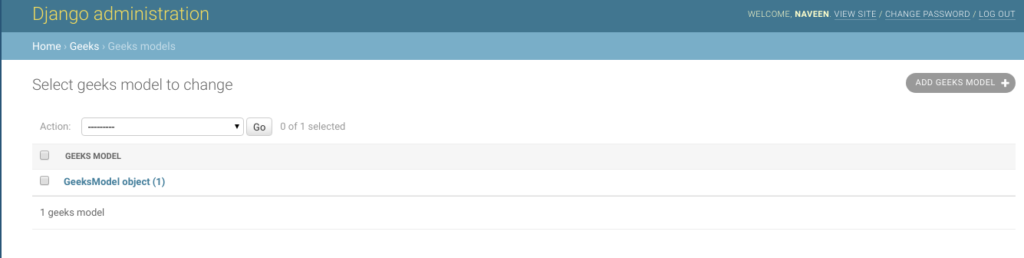Open In App

# DateField – Django Models

DateField is a field that stores date, represented in Python by a datetime.date instance. As the name suggests, this field is used to store an object of date created in python. The default form widget for this field is a TextInput. The admin can add a JavaScript calendar and a shortcut for “Today” explicitly.

Syntax:

`field_name = models.DateField(**options)`

DateField has the following extra optional arguments –

### DateField.auto_now

• Automatically set the field to now every time the object is saved. Useful for “last-modified” timestamps. Note that the current date is always used; it’s not just a default value that you can override.
The field is only automatically updated when calling Model.save(). The field isn’t updated when making updates to other fields in other ways such as QuerySet.update(), though you can specify a custom value for the field in an update like that.

• Automatically set the field to now when the object is first created. Useful for creation of timestamps. Note that the current date is always used; it’s not just a default value that you can override. So even if you set a value for this field when creating the object, it will be ignored. If you want to be able to modify this field, set the following instead of auto_now_add=True:
• For DateField: default=date.today – from datetime.date.today()
• For DateTimeField: default=timezone.now – from django.utils.timezone.now()

Note: The options auto_now_add, auto_now, and default are mutually exclusive. Any combination of these options will result in an error.

## Django Model DateField Explanation

Illustration of DateField using an Example. Consider a project named geeksforgeeks having an app named geeks.

Refer to the following articles to check how to create a project and an app in Django.

Enter the following code into the models.py file of geeks app.

## Python3

 `from` `django.db ``import` `models``from` `django.db.models ``import` `Model``# Create your models here.` `class` `GeeksModel(Model):``    ``geeks_field ``=` `models.DateField()`

Add the geeks app to INSTALLED_APPS

## Python3

 `# Application definition` `INSTALLED_APPS ``=` `[``    ``'django.contrib.admin'``,``    ``'django.contrib.auth'``,``    ``'django.contrib.contenttypes'``,``    ``'django.contrib.sessions'``,``    ``'django.contrib.messages'``,``    ``'django.contrib.staticfiles'``,``    ``'geeks'``,``]`

Now when we run makemigrations command from the terminal,

`Python manage.py makemigrations`

A new folder named migrations would be created in geeks directory with a file named 0001_initial.py

## Python3

 `# Generated by Django 2.2.5 on 2019-09-25 06:00` `from` `django.db ``import` `migrations, models` `class` `Migration(migrations.Migration):` `    ``initial ``=` `True` `    ``dependencies ``=` `[``    ``]` `    ``operations ``=` `[``        ``migrations.CreateModel(``            ``name ``=``'GeeksModel'``,``            ``fields ``=``[``                ``(``'id'``,``                  ``models.AutoField(``                  ``auto_created ``=` `True``,``                  ``primary_key ``=` `True``,``                  ``serialize ``=` `False``,``                  ``verbose_name ``=``'ID'``                ``)),``                ``(``'geeks_field'``, models.DateField()),``            ``],``        ``),``    ``]`

Now run,

`Python manage.py migrate`

Thus, an geeks_field DateField is created when you run migrations on the project. It is a field to store datetime.date python object.

## How to use DateField ?

DateField is used for storing python datetime.date instance in the database. One can store any type of date using the same in the database. Let’s try storing a date in model created above.

## Python3

 `# importing the model``# from geeks app``from` `geeks.models ``import` `GeeksModel` `# importing datetime module``import` `datetime` `# creating an instance of``# datetime.date``d ``=` `datetime.date(``1997``, ``10``, ``19``)` `# creating an instance of``# GeeksModel``geek_object ``=` `GeeksModel.objects.create(geeks_field ``=` `d)``geek_object.save()`

Now let’s check it in admin server. We have created an instance of GeeksModel.## Field Options

Field Options are the arguments given to each field for applying some constraint or imparting a particular characteristic to a particular Field. For example, adding an argument null = True to DateField will enable it to store empty values for that table in a relational database.
Here are the field options and attributes that a DateField can use.